# Boolean Algebra 1 L Alzaid Math 1101 Boolean

• Slides: 20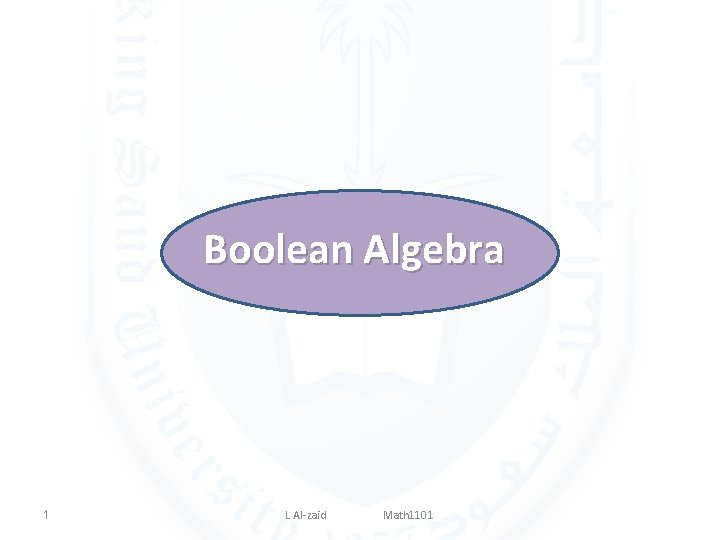Boolean Algebra 1 L Al-zaid Math 1101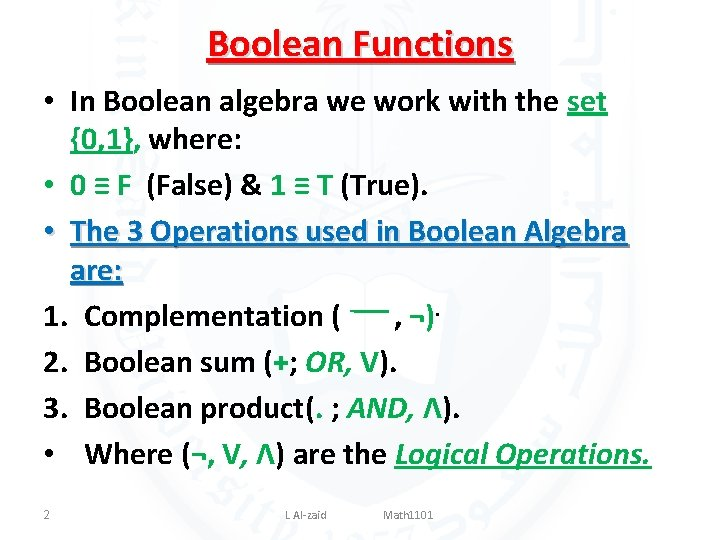Boolean Functions • In Boolean algebra we work with the set {0, 1}, where: • 0 ≡ F (False) & 1 ≡ T (True). • The 3 Operations used in Boolean Algebra are: 1. Complementation ( - ــــ , ¬). 2. Boolean sum (+; OR, V). 3. Boolean product(. ; AND, Λ). • Where (¬, V, Λ) are the Logical Operations. 2 L Al-zaid Math 1101The Operations In Boolean Algebra 1. The complementation of an element, denoted with a bar “- ”ــــ is defined by: 2. The sum (+; OR): 1+1=1; 1+0=1; 0+1=1; 0+0=0. 3. Boolean product(. ; AND). 1. 1=1, 1. 0=0, 0. 1=0, 0. 0=0 3 L Al-zaid Math 1101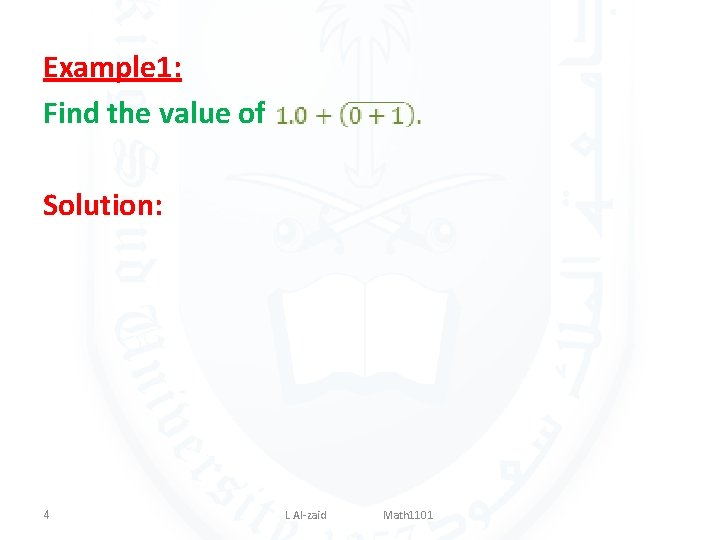Example 1: Find the value of Solution: 4 L Al-zaid Math 1101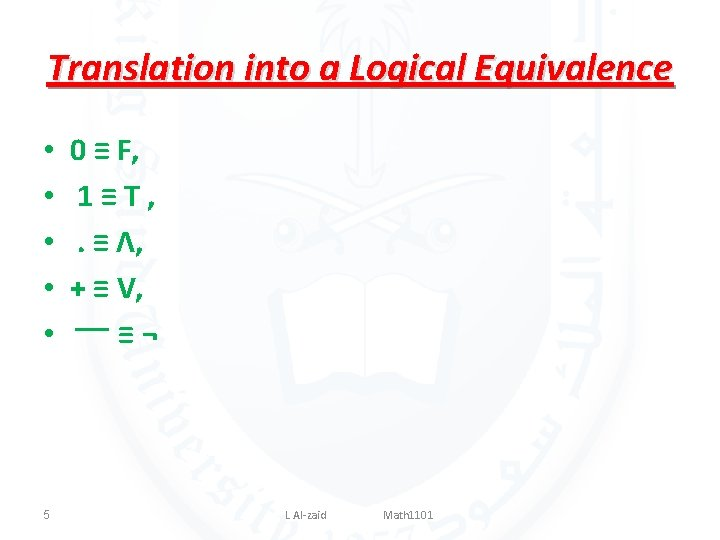Translation into a Logical Equivalence • • • 5 0 ≡ F, 1≡T, . ≡ Λ, + ≡ V, ¬ ≡ ــــ L Al-zaid Math 1101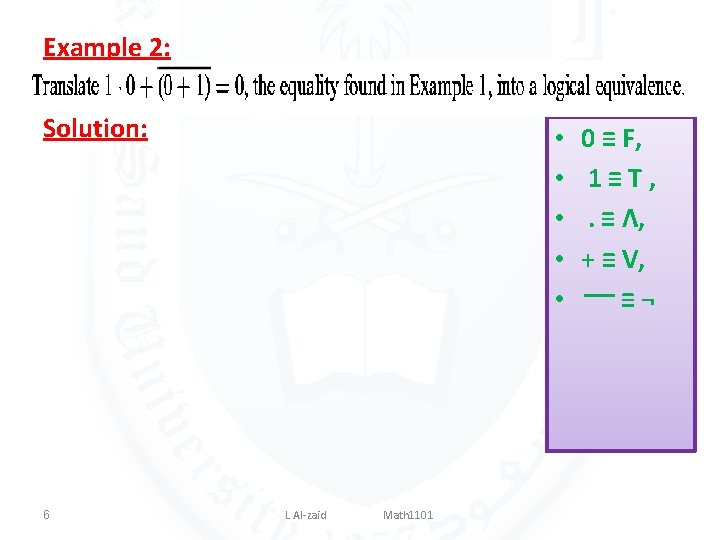Example 2: Solution: 6 • • • L Al-zaid Math 1101 0 ≡ F, 1≡T, . ≡ Λ, + ≡ V, ¬ ≡ ــــExample 3: • • • Solution: 7 L Al-zaid Math 1101 0 ≡ F, 1≡T, . ≡ Λ, + ≡ V, ¬ ≡ ــــ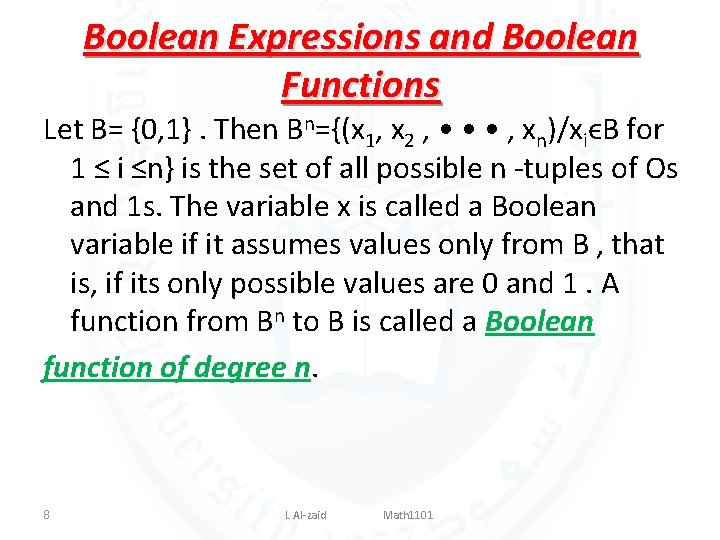Boolean Expressions and Boolean Functions Let B= {0, 1}. Then Bn={(x 1, x 2 , • • • , xn)/xiϵB for 1 ≤ i ≤n} is the set of all possible n -tuples of Os and 1 s. The variable x is called a Boolean variable if it assumes values only from B , that is, if its only possible values are 0 and 1. A function from Bn to B is called a Boolean function of degree n. 8 L Al-zaid Math 1101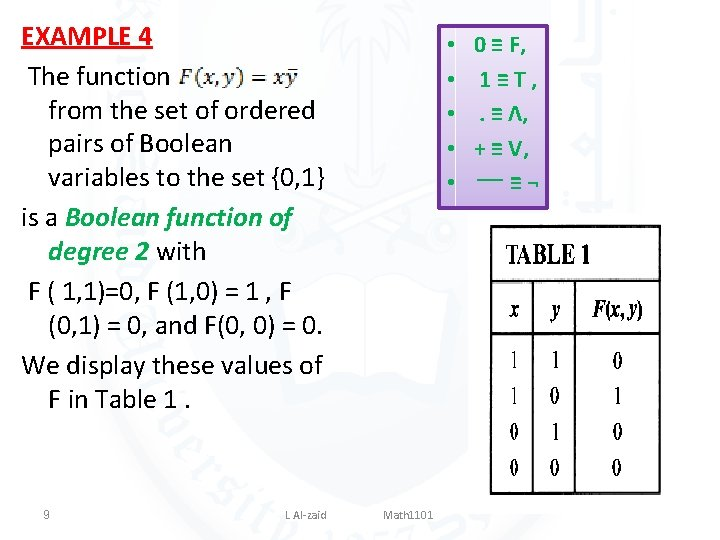EXAMPLE 4 The function from the set of ordered pairs of Boolean variables to the set {0, 1} is a Boolean function of degree 2 with F ( 1, 1)=0, F (1, 0) = 1 , F (0, 1) = 0, and F(0, 0) = 0. We display these values of F in Table 1. 9 L Al-zaid • • • Math 1101 0 ≡ F, 1≡T, . ≡ Λ, + ≡ V, ¬ ≡ ــــ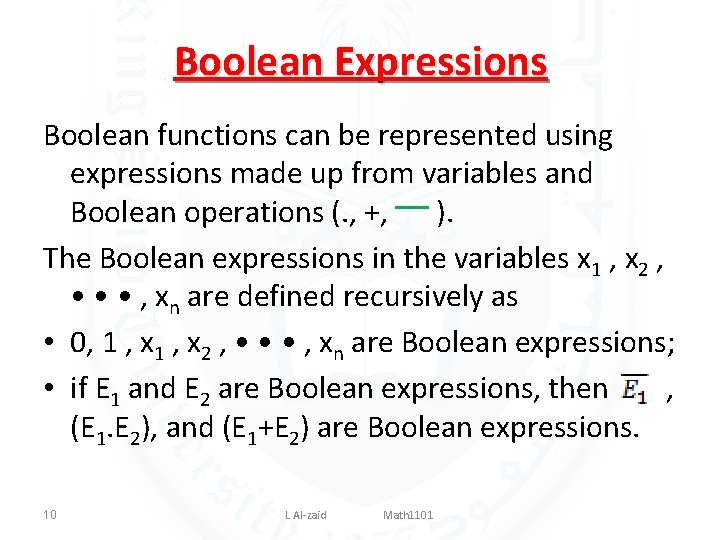Boolean Expressions Boolean functions can be represented using expressions made up from variables and Boolean operations (. , +, ) ــــ. The Boolean expressions in the variables x 1 , x 2 , • • • , xn are defined recursively as • 0, 1 , x 2 , • • • , xn are Boolean expressions; • if E 1 and E 2 are Boolean expressions, then , (E 1. E 2), and (E 1+E 2) are Boolean expressions. 10 L Al-zaid Math 1101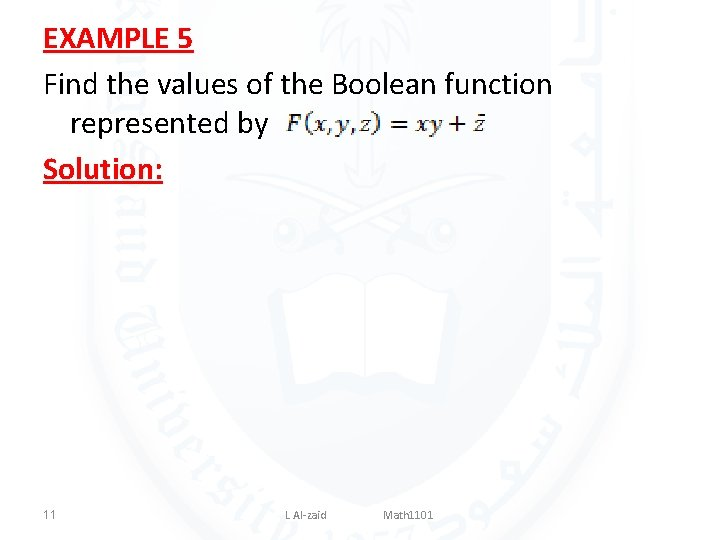EXAMPLE 5 Find the values of the Boolean function represented by Solution: 11 L Al-zaid Math 1101Equality of Boolean Functios 12 L Al-zaid Math 1101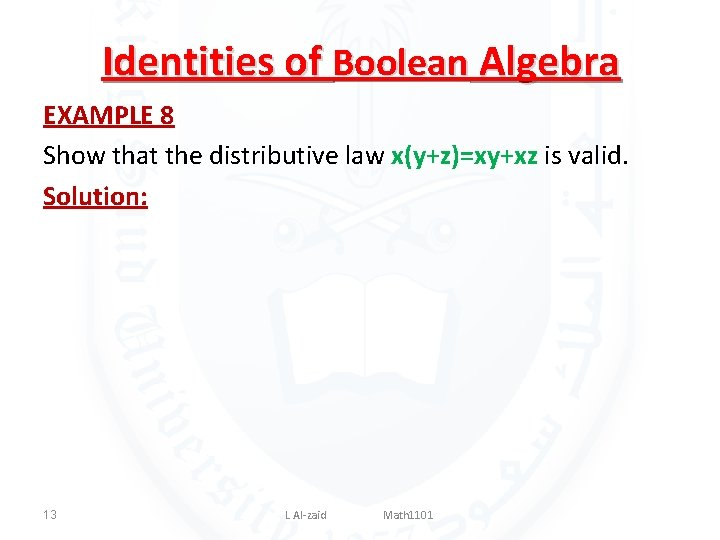Identities of Boolean Algebra EXAMPLE 8 Show that the distributive law x(y+z)=xy+xz is valid. Solution: 13 L Al-zaid Math 1101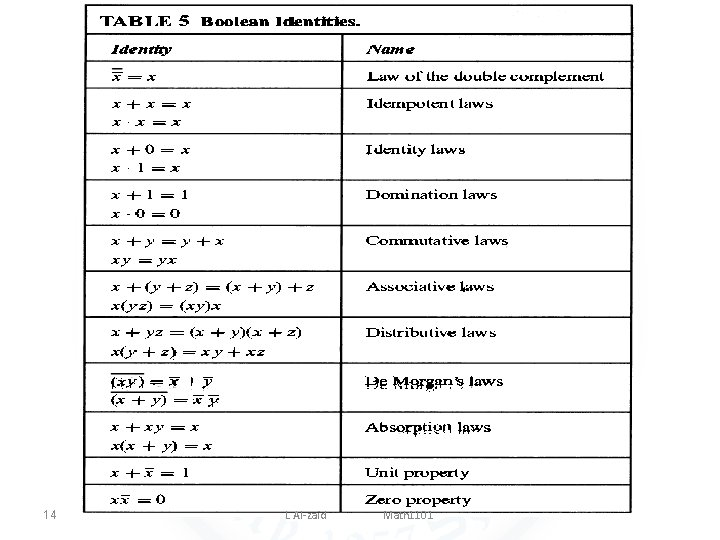14 L Al-zaid Math 1101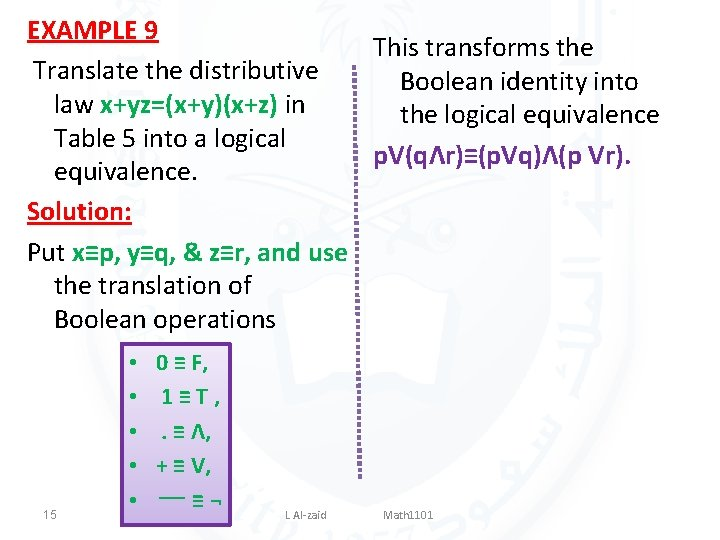EXAMPLE 9 This transforms the Translate the distributive Boolean identity into law x+yz=(x+y)(x+z) in the logical equivalence Table 5 into a logical p. V(qΛr)≡(p. Vq)Λ(p Vr). equivalence. Solution: Put x≡p, y≡q, & z≡r, and use the translation of Boolean operations 15 • • • 0 ≡ F, 1≡T, . ≡ Λ, + ≡ V, ¬ ≡ ــــ L Al-zaid Math 1101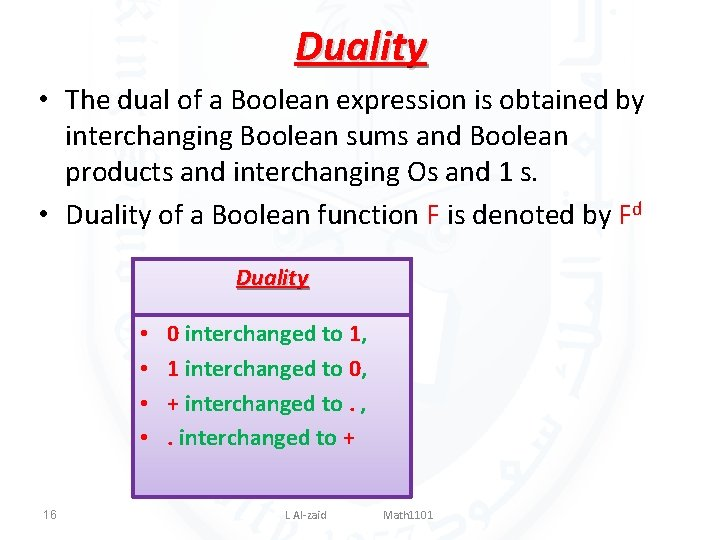Duality • The dual of a Boolean expression is obtained by interchanging Boolean sums and Boolean products and interchanging Os and 1 s. • Duality of a Boolean function F is denoted by Fd Duality • • 16 0 interchanged to 1, 1 interchanged to 0, + interchanged to. , . interchanged to + L Al-zaid Math 1101EXAMPLE 11 Solution: Duality • • 17 L Al-zaid Math 1101 0 interchanged to 1, 1 interchanged to 0, + interchanged to. , . interchanged to +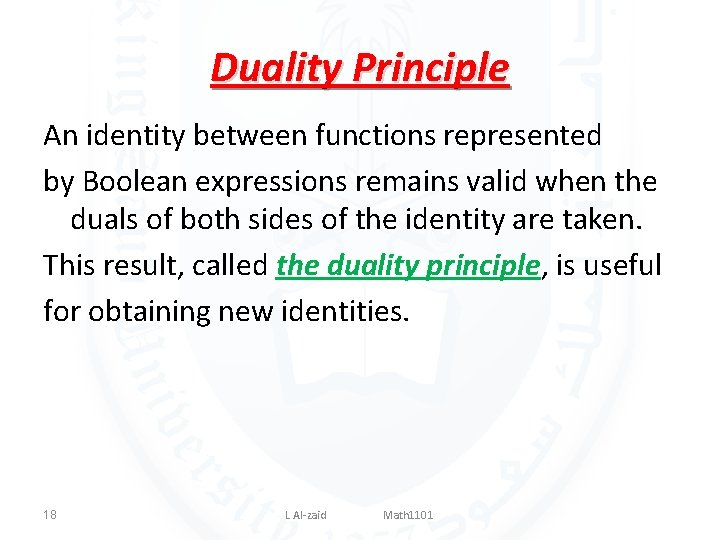Duality Principle An identity between functions represented by Boolean expressions remains valid when the duals of both sides of the identity are taken. This result, called the duality principle, is useful for obtaining new identities. 18 L Al-zaid Math 1101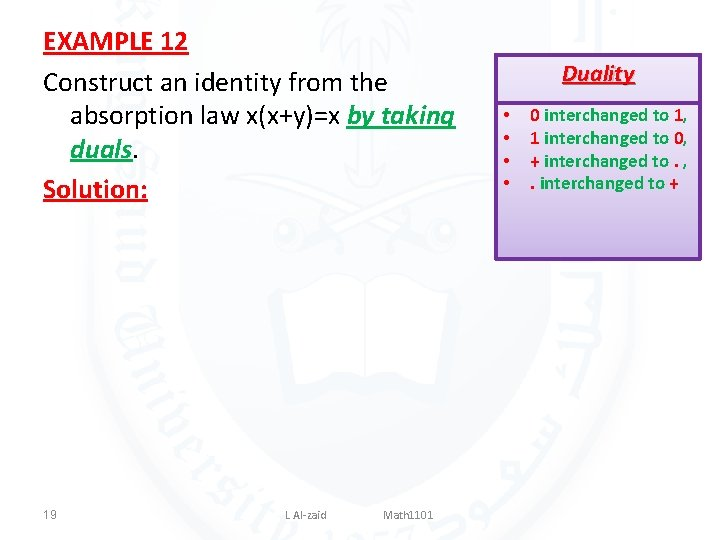EXAMPLE 12 Construct an identity from the absorption law x(x+y)=x by taking duals. Solution: 19 L Al-zaid Math 1101 Duality • • 0 interchanged to 1, 1 interchanged to 0, + interchanged to. , . interchanged to +Homework Page 756 • 1(a), • 4(a, b), • 5(a), • 11, • 28(a, d). 20 L Al-zaid Math 1101